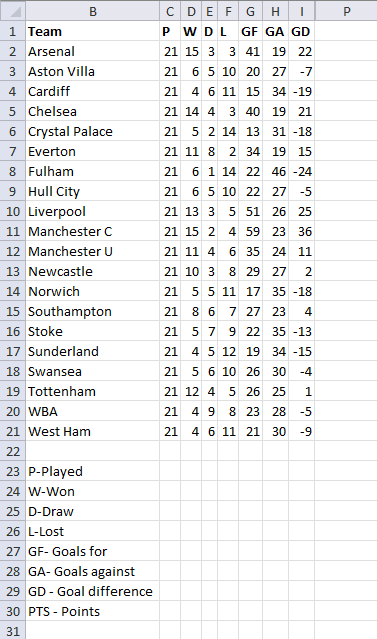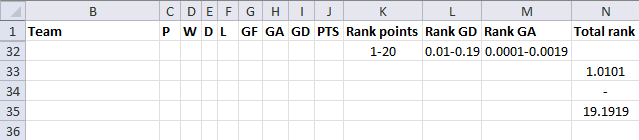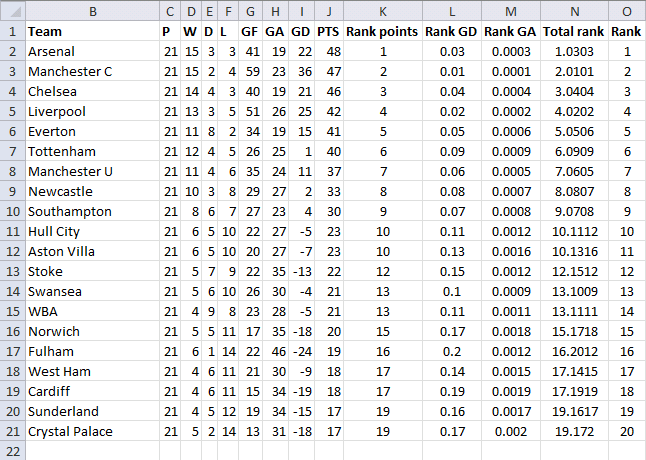Author: Oscar Cronquist Article last updated on July 30, 2017Today I´ll show you how to rank teams using excel formulas. The following example ranks soccer teams in the Premier League. But first, how are soccer teams ranked in Premier League?

Teams are ranked by total points, then goal difference, and then goals scored. At the end of each season, the club with the most points is crowned champion. If points are equal, the goal difference and then goals scored determine the winner. If still equal, teams are deemed to occupy the same position. Source: Wikipedia

The picture below shows teams and statistics in premier league, sorted by team name.### Calculating team ranks1. Teams are ranked by total points

Total points are calculated like this, a match winner gets 3 points, a draw gives 1 point. The formula in cell J2:

=D2*3+E2

Ranking points formula in cell K2:

=COUNTIF(\$J\$2:\$J\$21,">"&J2)+1

2. Teams are then ranked by goal difference

Ranking GD formula in cell L2:

=(COUNTIF(\$I\$2:\$I\$21,">"&I2)+1)/100

3. Teams are then ranked by goal score

Ranking GA formula in cell L2:

=(COUNTIF(\$G\$2:\$G\$21,">"&G2)+1)/10000

4. Sum rank values

Total rank in cell N2:

=K2+L2+M2

Calculating final rank in cell O2:

=COUNTIF(\$N\$2:\$N\$21,"<"&N2)+1

### Explaining formulas

The formulas are made so that the ranking calculations can be summed and the total rank shows the ranking order. For example, rank points are between 1-19. Rank Goal difference are between 0.01-0.19. Rank Goals against are between 0.0001 and 0.0019.The second formula is divided by 100 and the third formula is divided by 10000. So how do you know these numbers? What if the second formula was divided by 10? The numbers would be 0.1-1.9. Numbers equal to and above 1 distorts the first ranking formula. But if there were less than 10 teams the formula would return 0.1-0.9 and that is fine. Therefore, the calculations depend on the total number of teams.

If you want to know how the COUNTIF function works, read this: COUNTIF function

### Sort teams by rank

You can now sort the teams by rank. Select cell range B2:O21 and right click on range. Click "Sort" and then "Custom Sort...".Sort by Column O, smallest to largest order. Click OK.